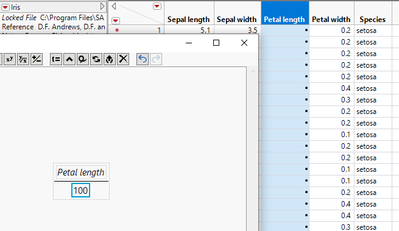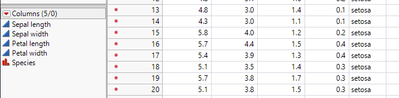Choose Language Hide Translation Bar
Highlighted

## How to modify(overwrite) existing column with formula in datatable

Hi,

I 'm new to JMP and would like to know how can I modify one of a column in my data table. Let's say we have iris data and would like to divide Petal length column / 100. I opened formula tab by right clicking Petal lenght and input my formula. In the end I got • in the column. What is the correct way to do this ?
thanks10 REPLIES 10
Highlighted

## Re: How to modify(overwrite) existing column with formula in datatable

Your formula column needs to go into a DIFFERENT column. You need to have the original data available in order to do the calculation. So, create a new column, maybe called Scaled Petal Width, and create the formula just as you have it now.

You can't overwrite the original column of data as that could cause a real conflict. Think of it this way. If you enter the formula in the column of original data, all of the values are divided by 100. But you still have a formula that says to change the entries to be divided by 100, so it should do that again. It would never stop. Further, displaying the original values along with the calculated values provides data integrity.

Now, if you REALLY want to overwrite, you can do so through scripting and is naturally more difficult. You would also not have the formula embedded in the column.

Dan Obermiller
Highlighted

## Re: How to modify(overwrite) existing column with formula in datatable

That's I don't want to do. In my real data table I have hundreds of columns and I just want to simply a simple math some of the. If I add more columns and have custom column names that increases the work I need to do! There should be I way around this.

Highlighted

## Re: How to modify(overwrite) existing column with formula in datatable

Here is one way to do that:

``````Names Default To Here( 1 );
dt = Current Data Table();

nameList = dt << get column names( string );

For( i = 1, i <= N Items( nameList ), i++,
If( Contains( nameList[i], "length" ),
For Each Row(
as Column( nameList[i] ) = as Column( nameList[i] ) / 100 )
)
);``````
Jim
Highlighted

## Re: How to modify(overwrite) existing column with formula in datatable

Using a formula to modify a column that has already existing static values will not work.  However, you can use JSL to modify the static values

``````For Each Row(
:Petal Length = :Petal Length/100;
);``````
Jim
Highlighted

## Re: How to modify(overwrite) existing column with formula in datatable

Where should I put this script? to formula window or somewhere else ?
Highlighted

## Re: How to modify(overwrite) existing column with formula in datatable

You put the script that Jim provided in a script window. Choose File > New > Script. Paste the script in there and run.

Dan Obermiller
Highlighted

## Re: How to modify(overwrite) existing column with formula in datatable

Wow! That worked. But it gave it to me 0's. ie 1.4/100 I think is not equal to 0.

Highlighted

## Re: How to modify(overwrite) existing column with formula in datatable

In addition how can I apply this formula to the columns contains certain strings. In this iris data set lets say 'length' is the string that I want to modify those column names that contains that.Highlighted

## Re: How to modify(overwrite) existing column with formula in datatable

Check the formatting of the column. More than likely the format is not set to "Best" and you are seeing rounded values.

Dan Obermiller
Article Labels

There are no labels assigned to this post.